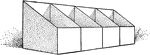### Capacity

"To find the number of bushels of grain in a bin or box, multiply the length in feet by the height in…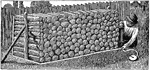### Cord Foot of Wood

"A pile of wood 1 ft. long, 4 ft. wide, and 4 ft. high, or 1/8 of a cord, is called a cord foot. Find…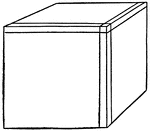### Cube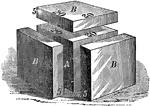A cube (A) has sides of 20 inches in length each, making its solid contents equal 8000 cubic inches.…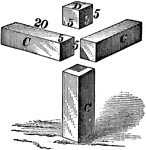In order to fill in the spaces from the three 2000 cubic inch additions, four new additions must be…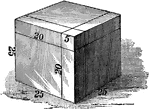This is the final form of the original 20x20x20 inch or 8000 cubic inch cube with the addition of 7625…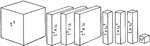### Cubing

A visual representation of cubing. "In the diagrams, Figure 1 represents 40 cubed and has a content…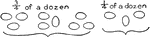### Dozen in Fourths

Visual of eggs showing 3/4 of a dozen and 1/4 of a dozen. 3x4=12, a dozen.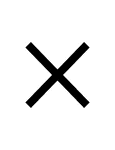### Flashcard of a math symbol for Multiplication

A flashcard featuring a math symbol for Multiplication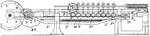### Multiplying or Dividing Machine

This machine is used for multiplication, and division purposes. Multiplication is the mathematical operation…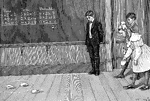### Math Game

Three students playing a math game in the classroom, throwing beanbags into a circle and doing multiplication…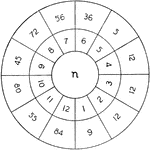### Multiples

The circle of multiples can be used as a practice game in class. The outside ring are multiples and…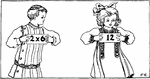### Children Holding Multiplication Cards

Illustration of 2 children comparing multiplication flash cards. It can be used to write mathematics…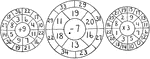### Number Wheels

Addition, subtraction, and multiplication wheels students use to spin and find the solutions.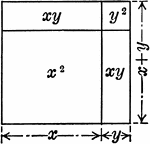### Representation of Algebraic Product of (x+y)(x+y)

An geometric illustration of the algebraic product of (x+y)(x+y).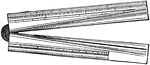### Sector

The sector is a hinged rule with scales used for various mathematical calculations.